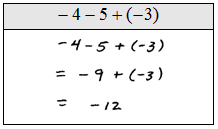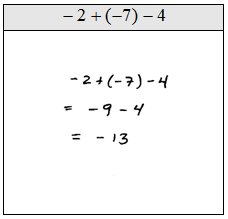## Pages

### Adding and Subtracting Integers

When first learning how to subtract real numbers, it is useful to think of this operation as adding opposite numbers. For example, 5 − 2 can be thought of as adding five with the opposite of two 5 + (−2). This may seem cumbersome, but sometimes it can help in understanding problems where you wish to subtract a negative number.Two sequential negative signs, as seen above, is equivalent to addition. When adding two negative numbers the result will be negative. For example, if you spend \$12.00 for a pair of shoes and \$25.00 for a bag then you will owe \$37.00 at checkout, −12 − 25 = −37.